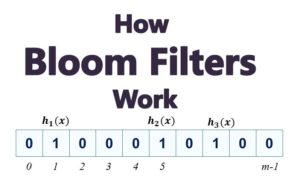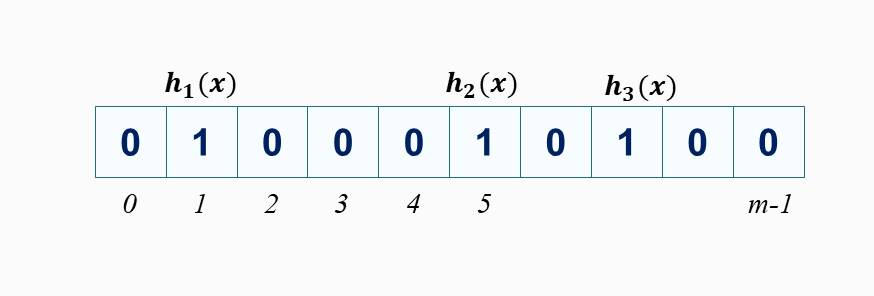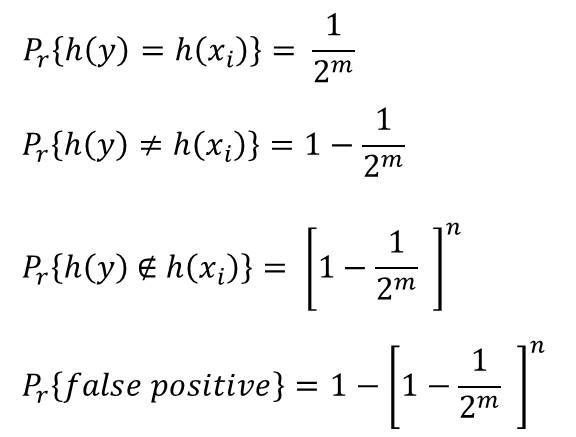# Bloom Filters – A Clear Explanation of How They Work

We would cover the following:1. Introduction to Bloom Filters

A Bloom Filter is a a data structure (based on hashing) that lets us determine whether an element is a member of a set. In bloom filters, it is possible for false positive to occur but with low probability. But false negatives is not possible. It is a space efficient and probabilistic data structure.

Bloom filters allows us to used several hash functions for each key.

2. Application of Bloom Filters

A common application of bloom filters ins in caching. The browser maintains certain levels of caches carefully placed to speed up loading of web pages that are accessed frequently. If a user requests a particular URL, then the browser needs to determine if the requested page is already in one of the caches and then serves the page from the cache if it is there.

However, it may happen that the page may the reported to be in the cache while it is actually not there. This is called false positive. In this case, then fetches he page from the original url.  Probability of this happening is small as we would see later.

3. How Bloom Filters Work

Remember that we mentioned that bloom filters are used to determine if an element is a member of a set.

So let’s assume we have a set of n elements S = {s1, s2, . . . , sn} which is taken from a very large universe U. (U may be a universe of all URLs and S is a set of urls existing in the cache). We want to allow for insertions into set S. Before insertion, the system checks if:

Given x  ∈ U, is x ∈   S?

• If the answer is No, then  x is not in S (x ∉ S)
• If the answer is Yes, then x may  or may not be in S

The objective is to perform this query as well as insertion into the cache in constant time O(1)

4. Bloom Filter Setup

A bloom filter is a bit array of B of m bits and k independent hash functions, h1, . . . , hk. The has functions map each key in U to a set Rm = {0, 1, . . . , m-1}

We assume that each of the hash function hi maps a uniformly random key  x ∈  U to each element of Rm with equal probability.

Since the hash functions h1, . . . , hk  are independent, then the result of the hashing, h1(x), . . . , hk(x) is equally likely to be any of the positions in Rm. All the m bits of B are initially set to 0. Therefore the process of insertion is as follows:

To Insert:  Evaluate  h1(x), . . . , hk(x) and set each of the bits to 1

To check is x ∈ S: Evaluate  h1(x), . . . , hk(x) . If all the bits are set to 1. then x ∈  S else x ∉ S

This is illustrated in Figure 1.0Figure 1.0: Bloom Filter. Initially all the bits are set to zero. Then we computer hk(x) and set the bits to 1 for each of the keys hk(x) maps to

5. Analysis of Bloom Filters – Version 1

In this version, we use k hash functions and k bit arrays.

To insert:

• h1(x) is stored in the first array
• h2(x is stored in the second array
• hk(x) is stored in the kth array

To search:

Search hi(x) for each i and if hi(x) is not set to 1 for any i, then x is not in the set

Let’s now calculate the probability of false positive

Pr(false positive) occurs if h(y) = h(xi)  where y ∉  S. Probability that this happens is given by:We can also go ahead to calculate the number of bits required.

6. Watch the Video

##### Check these Data Structure and Algorithms Tutorials#### kindsonthegenius

Kindson Munonye is currently completing his doctoral program in Software Engineering in Budapest University of Technology and Economics

View all posts by kindsonthegenius →